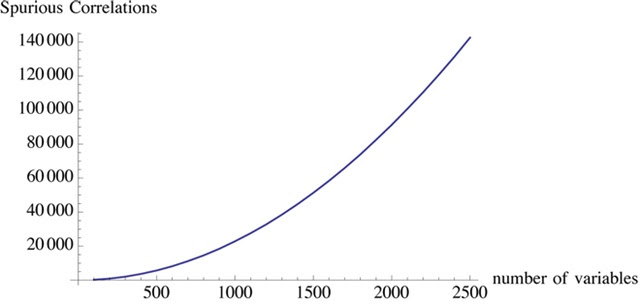## 05 junho 2019

### Paradoxo do Big Data: enganados pela aleatoriedade

Resumo:
Statisticians are increasingly posed with thought-provoking and even paradoxical questions, challenging our qualifications for entering the statistical paradises created by Big Data. By developing measures for data quality, this article suggests a framework to address such a question: “Which one should I trust more: a 1% survey with 60% response rate or a self-reported administrative dataset covering 80% of the population?” A 5-element Euler-formula-like identity shows that for any dataset of size $n$, probabilistic or not, the difference between the sample average ${\overline{X}}_{n}$and the population average ${\overline{X}}_{N}$ is the product of three terms: (1) a data quality measure, ${\rho }_{R,X}$, the correlation between ${X}_{j}$ and the response/recording indicator ${R}_{j}$; (2) a data quantity measure, $\sqrt{\left(N-n\right)/n}$, where $N$ is the population size; and (3) a problem difficultymeasure, ${\sigma }_{X}$, the standard deviation of $X$. This decomposition provides multiple insights: (I) Probabilistic sampling ensures high data quality by controlling ${\rho }_{R,X}$ at the level of ${N}^{-1/2}$; (II) When we lose this control, the impact of $N$ is no longer canceled by ${\rho }_{R,X}$, leading to a Law of Large Populations (LLP), that is, our estimation error, relative to the benchmarking rate $1/\sqrt{n}$, increases with $\sqrt{N}$; and (III) the “bigness” of such Big Data (for population inferences) should be measured by the relative size $f=n/N$, not the absolute size $n$; (IV) When combining data sources for population inferences, those relatively tiny but higher quality ones should be given far more weights than suggested by their sizes.
Estimates obtained from the Cooperative Congressional Election Study (CCES) of the 2016 US presidential election suggest a ${\rho }_{R,X}\approx -0.005$for self-reporting to vote for Donald Trump. Because of LLP, this seemingly minuscule data defect correlation implies that the simple sample proportion of the self-reported voting preference for Trump from $1\mathrm{%}$ of the US eligible voters, that is, $n\approx 2\text{,}300\text{,}000$, has the same mean squared error as the corresponding sample proportion from a genuine simple random sample of size $n\approx 400$, a $99.98\mathrm{%}$ reduction of sample size (and hence our confidence). The CCES data demonstrate LLP vividly: on average, the larger the state’s voter populations, the further away the actual Trump vote shares from the usual $95\mathrm{%}$ confidence intervals based on the sample proportions. This should remind us that, without taking data quality into account, population inferences with Big Data are subject to a Big Data Paradox: the more the data, the surer we fool ourselves.
Fonte:
Meng, Xiao-Li. Statistical paradises and paradoxes in big data (I): Law of large populations, big data paradox, and the 2016 US presidential election. Ann. Appl. Stat. 12 (2018), no. 2, 685--726. doi:10.1214/18-AOAS1161SF. https://projecteuclid.org/euclid.aoas/1532743473